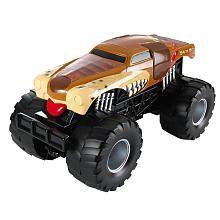# Hot Wheels Monster Jam Truck Monster Mutt Sound Smashers

Vendor
Hot Wheels
Regular price
Sold out
Sale price
\$95.54
Quantity must be 1 or more

• H

• o

• t

• W

• h

• e

• e

• l

• s

• M

• o

• n

• s

• t

• e

• r

• J

• a

• m

• T

• r

• u

• c

• k

• M

• o

• n

• s

• t

• e

• r

• M

• u

• t

• t

• S

• o

• u

• n

• d

• S

• m

• a

• s

• h

• e

• r

• s

Hot Wheels Monster Jam Truck, little bigger than 1:24 scale truck, three ways to make sounds.